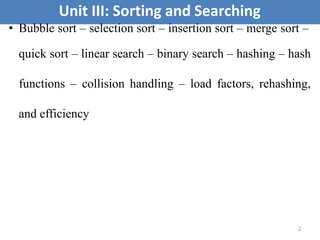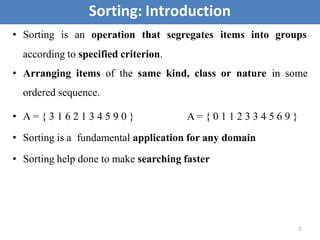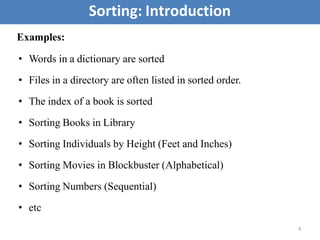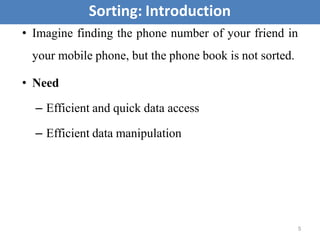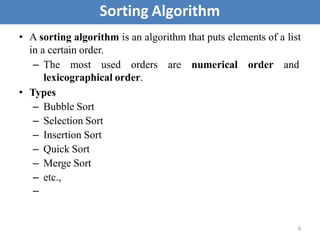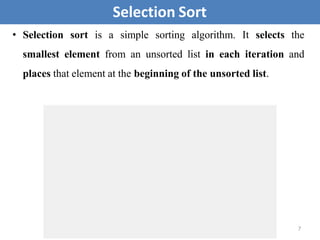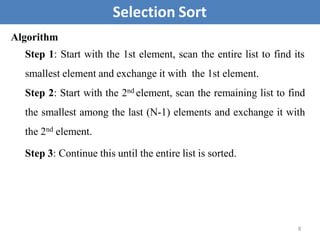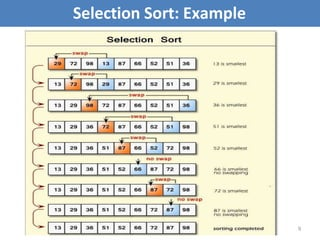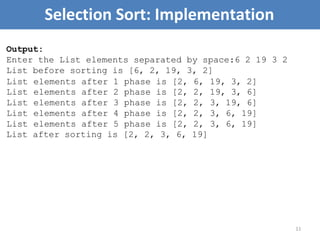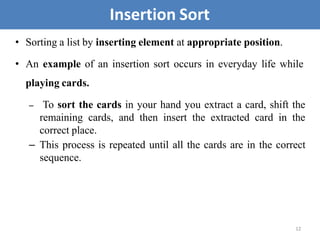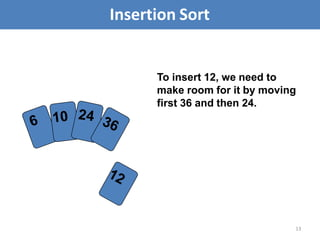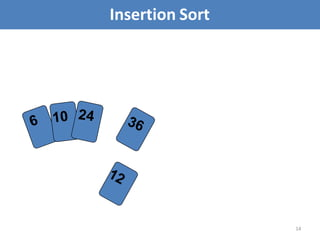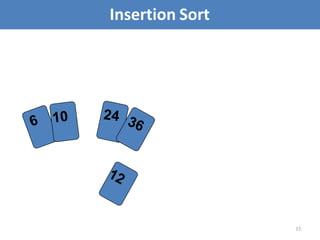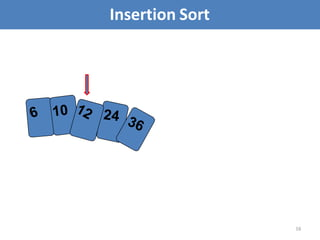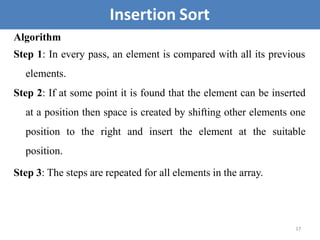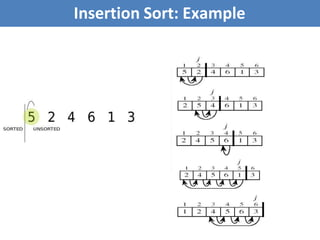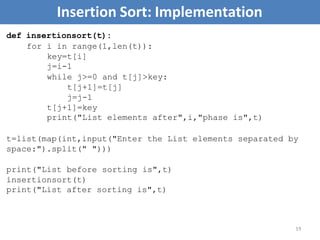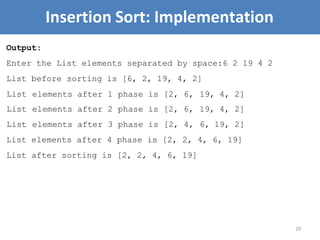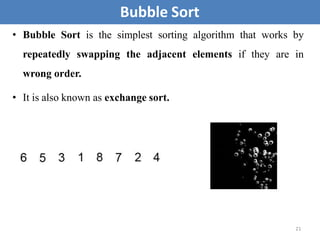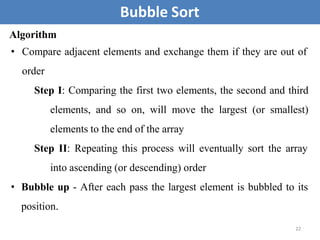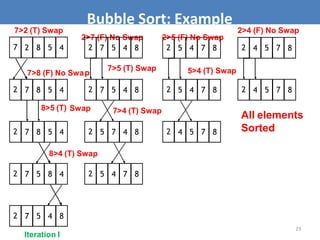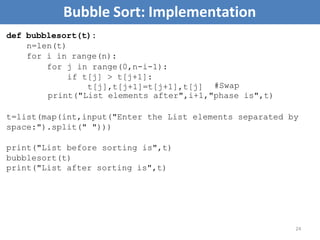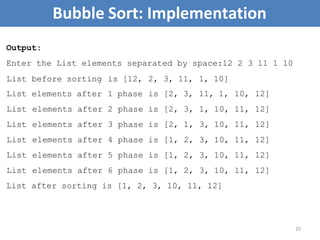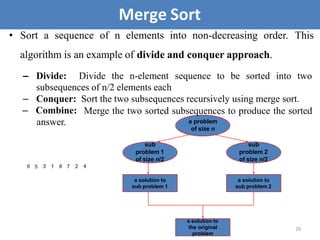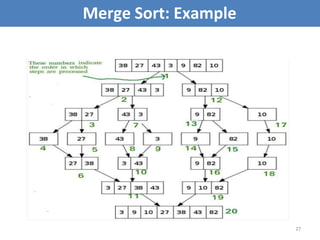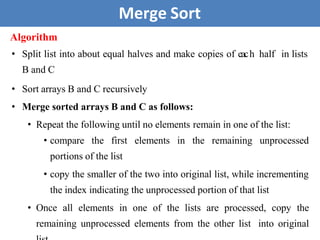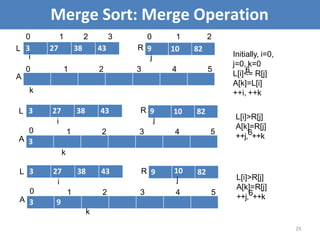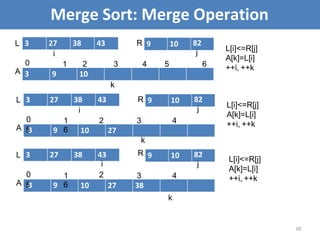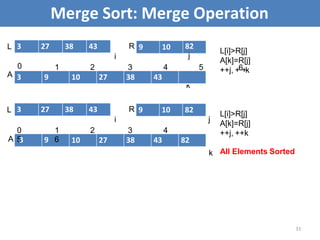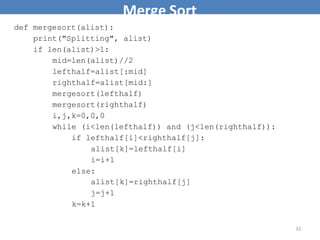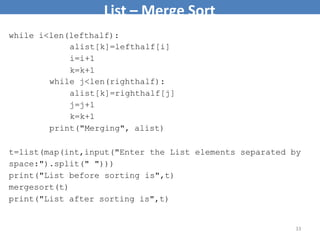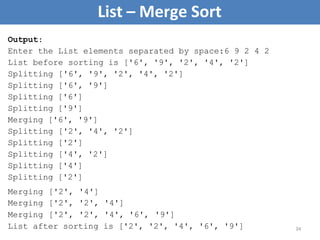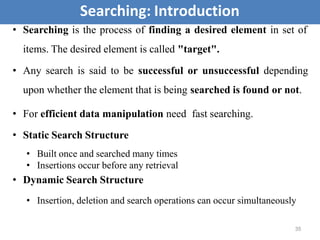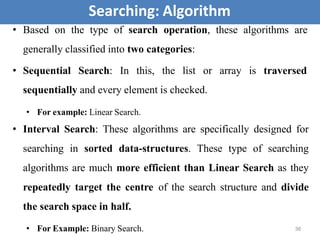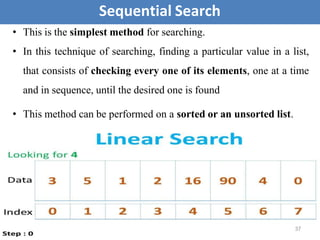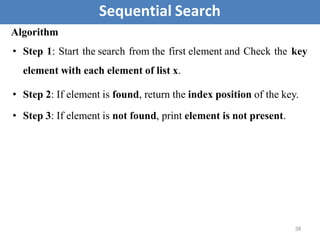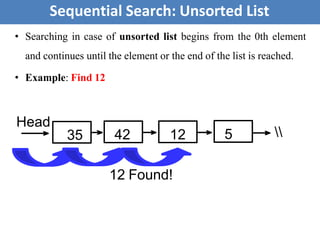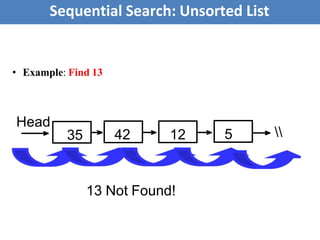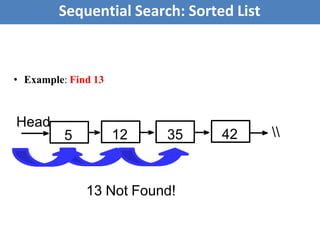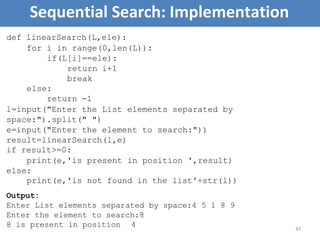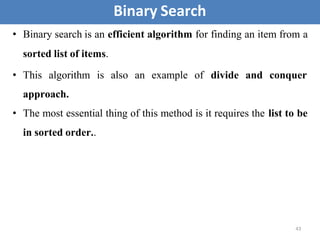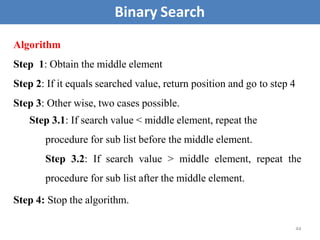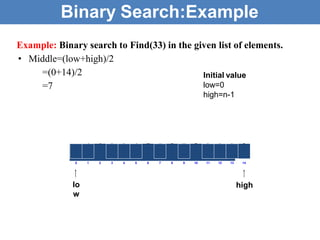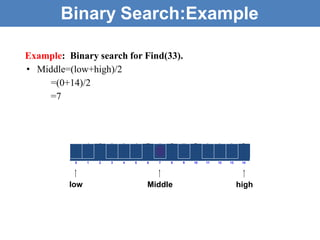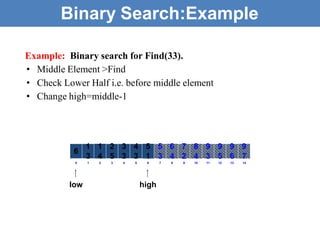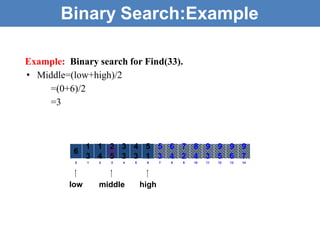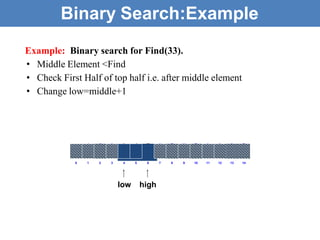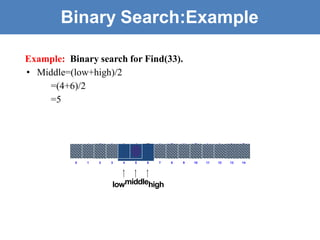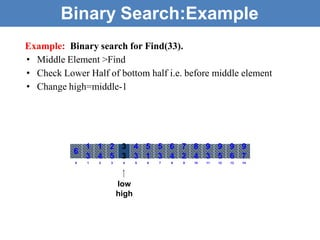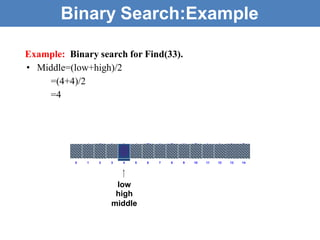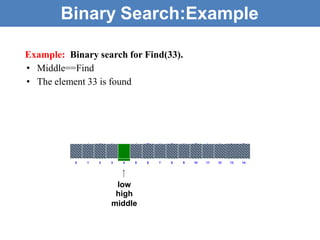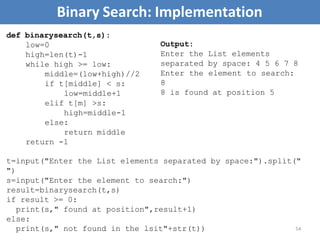1 of 54

### Unit III Version I.pptx

1. 1 AD8251 - DATA STRUCTURES DESIGN Unit III: SORTING AND SEARCHING
2. Unit III: Sorting and Searching 2 • Bubble sort – selection sort – insertion sort – merge sort – quick sort – linear search – binary search – hashing – hash functions – collision handling – load factors, rehashing, and efficiency
3. • Sorting is an operation that segregates items into groups according to specified criterion. • Arranging items of the same kind, class or nature in some ordered sequence. • A = { 3 1 6 2 1 3 4 5 9 0 } A = { 0 1 1 2 3 3 4 5 6 9 } • Sorting is a fundamental application for any domain • Sorting help done to make searching faster Sorting: Introduction 3
4. Examples: • Words in a dictionary are sorted • Files in a directory are often listed in sorted order. • The index of a book is sorted • Sorting Books in Library • Sorting Individuals by Height (Feet and Inches) • Sorting Movies in Blockbuster (Alphabetical) • Sorting Numbers (Sequential) • etc Sorting: Introduction 4
5. • Imagine finding the phone number of your friend in your mobile phone, but the phone book is not sorted. • Need – Efficient and quick data access – Efficient data manipulation Sorting: Introduction 5
6. • A sorting algorithm is an algorithm that puts elements of a list in a certain order. – The most used orders lexicographical order. • Types – Bubble Sort – Selection Sort – Insertion Sort – Quick Sort – Merge Sort – etc., are numerical order and – Sorting Algorithm 6
7. Selection Sort • Selection sort is a simple sorting algorithm. It selects the smallest element from an unsorted list in each iteration and places that element at the beginning of the unsorted list. 7
8. Selection Sort 8 Algorithm Step 1: Start with the 1st element, scan the entire list to find its smallest element and exchange it with the 1st element. Step 2: Start with the 2nd element, scan the remaining list to find the smallest among the last (N-1) elements and exchange it with the 2nd element. Step 3: Continue this until the entire list is sorted.
9. Selection Sort: Example 9
10. 10 Selection Sort: Implementation def selectionsort(t): #Method I: for i in range(len(t)): min_index=i for j in range(i+1,len(t)): if t[j] < t[min_index]: min_index=j t[min_index],t[i]=t[i],t[min_index] print("List elements after",i+1,"phase is",t) def selectionsort(t): #Method II: for i in range(len(t)): smallest=min(t[i:]) index_of_smallest=t.index(smallest,i) t[i],t[index_of_smallest]=t[index_of_smallest],t[i] print("List elements after",i+1,"phase is",t) t=list(map(int,input("Enter the List elements separated by space:").split(" "))) print("List before sorting is",t) selectionsort(t) print("List after sorting is",t)
11. Selection Sort: Implementation 11 Output: Enter the List elements separated by space:6 2 19 3 2 List before sorting is [6, 2, 19, 3, 2] List elements after 1 phase is [2, 6, 19, 3, 2] List elements after 2 phase is [2, 2, 19, 3, 6] List elements after 3 phase is [2, 2, 3, 19, 6] List elements after 4 phase is [2, 2, 3, 6, 19] List elements after 5 phase is [2, 2, 3, 6, 19] List after sorting is [2, 2, 3, 6, 19]
12. • Sorting a list by inserting element at appropriate position. • An example of an insertion sort occurs in everyday life while playing cards. – To sort the cards in your hand you extract a card, shift the remaining cards, and then insert the extracted card in the correct place. – This process is repeated until all the cards are in the correct sequence. Insertion Sort 12
13. To insert 12, we need to make room for it by moving first 36 and then 24. Insertion Sort 13
14. Insertion Sort 14
15. Insertion Sort 15
16. Insertion Sort 16
17. Algorithm Step 1: In every pass, an element is compared with all its previous elements. Step 2: If at some point it is found that the element can be inserted at a position then space is created by shifting other elements one position to the right and insert the element at the suitable position. Step 3: The steps are repeated for all elements in the array. Insertion Sort 17
18. Insertion Sort: Example
19. Insertion Sort: Implementation 19 def insertionsort(t): for i in range(1,len(t)): key=t[i] j=i-1 while j>=0 and t[j]>key: t[j+1]=t[j] j=j-1 t[j+1]=key print("List elements after",i,"phase is",t) t=list(map(int,input("Enter the List elements separated by space:").split(" "))) print("List before sorting is",t) insertionsort(t) print("List after sorting is",t)
20. Insertion Sort: Implementation 20 Output: Enter the List elements separated by space:6 2 19 4 2 List before sorting is [6, 2, 19, 4, 2] List elements after 1 phase is [2, 6, 19, 4, 2] List elements after 2 phase is [2, 6, 19, 4, 2] List elements after 3 phase is [2, 4, 6, 19, 2] List elements after 4 phase is [2, 2, 4, 6, 19] List after sorting is [2, 2, 4, 6, 19]
21. Bubble Sort • Bubble Sort is the simplest sorting algorithm that works by repeatedly swapping the adjacent elements if they are in wrong order. • It is also known as exchange sort. 21
22. Algorithm • Compare adjacent elements and exchange them if they are out of order Step I: Comparing the first two elements, the second and third elements, and so on, will move the largest (or smallest) elements to the end of the array Step II: Repeating this process will eventually sort the array into ascending (or descending) order • Bubble up - After each pass the largest element is bubbled to its position. Bubble Sort 22
23. 7 2 8 5 4 2 7 8 5 4 8>5 (T) 2 7 8 5 4 2 7 5 8 4 2 7 5 4 8 2 5 7 4 8 2 5 4 7 8 2 7 5 4 8 2 5 4 7 8 2 4 5 7 8 2 5 4 7 8 2 4 5 7 8 2 4 5 7 8 All elements Sorted 23 Bubble Sort: Example 2>7 (F) No Swap 2>5 (F) No Swap 7>2 (T) Swap 7>8 (F) No Swa 2 7 5 4 8 p Swap 8>4 (T) Swap 7>5 (T) Swap 7>4 (T) Swap 5>4 (T) Swap 2>4 (F) No Swap Iteration I
24. Bubble Sort: Implementation 24 def bubblesort(t): n=len(t) for i in range(n): for j in range(0,n-i-1): if t[j] > t[j+1]: t[j],t[j+1]=t[j+1],t[j] #Swap print("List elements after",i+1,"phase is",t) t=list(map(int,input("Enter the List elements separated by space:").split(" "))) print("List before sorting is",t) bubblesort(t) print("List after sorting is",t)
25. Bubble Sort: Implementation 25 Output: Enter the List elements separated by space:12 2 3 11 1 10 List before sorting is [12, 2, 3, 11, 1, 10] List elements after 1 phase is [2, 3, 11, 1, 10, 12] List elements after 2 phase is [2, 3, 1, 10, 11, 12] List elements after 3 phase is [2, 1, 3, 10, 11, 12] List elements after 4 phase is [1, 2, 3, 10, 11, 12] List elements after 5 phase is [1, 2, 3, 10, 11, 12] List elements after 6 phase is [1, 2, 3, 10, 11, 12] List after sorting is [1, 2, 3, 10, 11, 12]
26. • Sort a sequence of n elements into non-decreasing order. This algorithm is an example of divide and conquer approach. – Divide: Divide the n-element sequence to be sorted into two subsequences of n/2 elements each – Conquer: Sort the two subsequences recursively using merge sort. – Combine: answer. 26 Merge Sort sub problem 2 of size n/2 sub problem 1 of size n/2 a solution to sub problem 1 a solution to the original problem a solution to sub problem 2 Merge the two sorted subsequences to produce the sorted a problem of size n
27. 27 Merge Sort: Example
28. Algorithm • Split list into about equal halves and make copies of eac2h8 half in lists B and C • Sort arrays B and C recursively • Merge sorted arrays B and C as follows: • Repeat the following until no elements remain in one of the list: • compare the first elements in the remaining unprocessed portions of the list • copy the smaller of the two into original list, while incrementing the index indicating the unprocessed portion of that list • Once all elements in one of the lists are processed, copy the remaining unprocessed elements from the other list into original Merge Sort
29. 3 27 38 43 10 82 R 9 L 0 1 2 3 0 1 2 i j A 0 1 2 3 4 5 k Initially, i=0, j=0, k=0 L[i]< 6 = R[j] A[k]=L[i] ++i, ++k 3 27 38 43 10 82 R 9 L i j 3 0 A 1 2 3 4 5 k L[i]>R[j] A[k]=R[j] ++j,6++k 3 27 38 43 9 82 10 j L R i 3 9 0 A 1 2 3 4 5 k L[i]>R[j] A[k]=R[j] ++j,6++k Merge Sort: Merge Operation 29
30. k L[i]<=R[j] A[k]=L[i] ++i, ++k 3 27 38 43 9 10 82 j L R i 3 9 10 0 A 1 2 3 4 5 6 L[i]<=R[j] A[k]=L[i] ++i, ++k 3 27 38 43 9 10 82 j L R i 0 A 1 2 3 4 5 3 9 6 10 27 k 3 27 38 43 9 10 k R 82 j L 0 A 1 i 2 3 4 5 3 9 6 10 27 38 L[i]<=R[j] A[k]=L[i] ++i, ++k Merge Sort: Merge Operation 30
31. k 3 27 38 43 9 10 82 j L R i 3 9 10 27 38 43 0 A 1 2 3 4 5 L[i]>R[j] A[k]=R[j] ++j, +6+k k 3 27 38 43 9 10 82 R i j 0 1 2 3 4 5 3 9 6 10 27 38 43 82 L[i]>R[j] A[k]=R[j] ++j, ++k All Elements Sorted Merge Sort: Merge Operation 31 L A
32. Merge Sort 32 def mergesort(alist): print("Splitting", alist) if len(alist)>1: mid=len(alist)//2 lefthalf=alist[:mid] righthalf=alist[mid:] mergesort(lefthalf) mergesort(righthalf) i,j,k=0,0,0 while (i<len(lefthalf)) and (j<len(righthalf)): if lefthalf[i]<righthalf[j]: alist[k]=lefthalf[i] i=i+1 else: alist[k]=righthalf[j] j=j+1 k=k+1
33. List – Merge Sort 33 while i<len(lefthalf): alist[k]=lefthalf[i] i=i+1 k=k+1 while j<len(righthalf): alist[k]=righthalf[j] j=j+1 k=k+1 print("Merging", alist) t=list(map(int,input("Enter the List elements separated by space:").split(" "))) print("List before sorting is",t) mergesort(t) print("List after sorting is",t)
34. 34 List – Merge Sort Output: Enter the List elements separated by space:6 9 2 4 2 List before sorting is ['6', '9', '2', '4', '2'] Splitting ['6', '9', '2', '4', '2'] Splitting ['6', '9'] Splitting ['6'] Splitting ['9'] Merging ['6', '9'] Splitting ['2', '4', '2'] Splitting ['2'] Splitting ['4', '2'] Splitting ['4'] Splitting ['2'] Merging ['2', '4'] Merging ['2', '2', '4'] Merging ['2', '2', '4', '6', '9'] List after sorting is ['2', '2', '4', '6', '9']
35. 35 • Searching is the process of finding a desired element in set of items. The desired element is called "target". • Any search is said to be successful or unsuccessful depending upon whether the element that is being searched is found or not. • For efficient data manipulation need fast searching. • Static Search Structure • Built once and searched many times • Insertions occur before any retrieval • Dynamic Search Structure • Insertion, deletion and search operations can occur simultaneously Searching: Introduction
36. 36 • Based on the type of search operation, these algorithms are generally classified into two categories: • Sequential Search: In this, the list or array is traversed sequentially and every element is checked. • For example: Linear Search. • Interval Search: These algorithms are specifically designed for searching in sorted data-structures. These type of searching algorithms are much more efficient than Linear Search as they repeatedly target the centre of the search structure and divide the search space in half. • For Example: Binary Search. Searching: Algorithm
37. • This is the simplest method for searching. • In this technique of searching, finding a particular value in a list, that consists of checking every one of its elements, one at a time and in sequence, until the desired one is found • This method can be performed on a sorted or an unsorted list. Sequential Search 37
38. Algorithm • Step 1: Start the search from the first element and Check the key element with each element of list x. • Step 2: If element is found, return the index position of the key. • Step 3: If element is not found, print element is not present. Sequential Search 38
39. • Searching in case of unsorted list begins from the 0th element and continues until the element or the end of the list is reached. • Example: Find 12 35 42 12 5 12 Found! Head Sequential Search: Unsorted List
40. • Example: Find 13 35 42 12 5 Head Sequential Search: Unsorted List 13 Not Found!
41. • Example: Find 13 5 12 35 42 Head Sequential Search: Sorted List 13 Not Found!
42. 42 Sequential Search: Implementation def linearSearch(L,ele): for i in range(0,len(L)): if(L[i]==ele): return i+1 break else: return -1 l=input("Enter the List elements separated by space:").split(" ") e=input("Enter the element to search:")) result=linearSearch(l,e) if result>=0: print(e,'is present in position ',result) else: print(e,'is not found in the list'+str(l)) Output: Enter List elements separated by space:4 5 1 8 9 Enter the element to search:8 8 is present in position 4
43. • Binary search is an efficient algorithm for finding an item from a sorted list of items. divide and conquer • This algorithm is also an example of approach. • The most essential thing of this method is it requires the list to be in sorted order.. Binary Search 43
44. Binary Search 44 Algorithm Step 1: Obtain the middle element Step 2: If it equals searched value, return position and go to step 4 Step 3: Other wise, two cases possible. Step 3.1: If search value < middle element, repeat the procedure for sub list before the middle element. Step 3.2: If search value > middle element, repeat the procedure for sub list after the middle element. Step 4: Stop the algorithm.
45. 6 1 3 1 4 2 5 3 3 4 3 5 1 5 3 6 4 7 2 8 4 9 3 9 5 9 6 9 7 0 lo w 1 2 3 4 5 6 7 8 9 10 11 12 13 14 high Example: Binary search to Find(33) in the given list of elements. • Middle=(low+high)/2 =(0+14)/2 =7 Binary Search:Example Initial value low=0 high=n-1
46. Example: Binary search for Find(33). • Middle=(low+high)/2 =(0+14)/2 =7 6 1 3 1 4 2 5 3 3 4 3 5 1 5 3 6 4 7 2 8 4 9 3 9 5 9 6 9 7 0 1 2 3 4 5 6 7 8 9 10 11 12 13 14 low high Middle Binary Search:Example
47. Example: Binary search for Find(33). • Middle Element >Find • Check Lower Half i.e. before middle element • Change high=middle-1 1 1 2 3 4 5 5 6 7 8 9 9 9 9 6 3 4 5 3 3 1 3 4 2 4 3 5 6 7 0 low 1 2 3 4 5 6 high 7 8 9 10 11 12 13 14 Binary Search:Example
48. Example: Binary search for Find(33). • Middle=(low+high)/2 =(0+6)/2 =3 1 1 2 3 4 5 5 6 7 8 9 9 9 9 3 4 5 3 3 1 3 4 2 4 3 5 6 7 0 1 2 3 4 5 6 7 8 9 10 11 12 13 14 6 low middle high Binary Search:Example
49. Example: Binary search for Find(33). • Middle Element <Find • Check First Half of top half i.e. after middle element • Change low=middle+1 6 1 3 1 4 2 5 3 3 4 3 5 1 5 3 6 4 7 2 8 4 9 3 9 5 9 6 9 7 0 1 2 3 4 low 5 6 high 7 8 9 10 11 12 13 14 Binary Search:Example
50. Example: Binary search for Find(33). • Middle=(low+high)/2 =(4+6)/2 =5 6 1 3 1 4 2 5 3 3 4 3 5 1 5 3 6 4 7 2 8 4 9 3 9 5 9 6 9 7 0 1 2 3 4 5 6 7 8 9 10 11 12 13 14 lowmiddlehigh Binary Search:Example
51. Example: Binary search for Find(33). • Middle Element >Find • Check Lower Half of bottom half i.e. before middle element • Change high=middle-1 8 2 1 3 4 6 5 7 10 9 11 12 14 13 0 1 1 2 3 4 5 5 6 7 8 9 9 9 9 3 4 5 3 3 1 3 4 2 4 3 5 6 7 6 low high Binary Search:Example
52. Example: Binary search for Find(33). • Middle=(low+high)/2 =(4+4)/2 =4 6 1 3 1 4 2 5 3 3 4 3 5 1 5 3 6 4 7 2 8 4 9 3 9 5 9 6 9 7 0 1 2 3 4 5 6 7 8 9 10 11 12 13 14 Binary Search:Example low high middle
53. Example: Binary search for Find(33). • Middle==Find • The element 33 is found 6 1 3 1 4 2 5 3 3 4 3 5 1 5 3 6 4 7 2 8 4 9 3 9 5 9 6 9 7 0 1 2 3 4 5 6 7 8 9 10 11 12 13 14 Binary Search:Example low high middle
54. 54 Binary Search: Implementation def binarysearch(t,s): low=0 high=len(t)-1 while high >= low: middle=(low+high)//2 if t[middle] < s: low=middle+1 elif t[m] >s: high=middle-1 else: return middle return -1 t=input("Enter the List elements separated by space:").split(" ") s=input("Enter the element to search:") result=binarysearch(t,s) if result >= 0: print(s," found at position",result+1) else: print(s," not found in the lsit"+str(t)) Output: Enter the List elements separated by space: 4 5 6 7 8 Enter the element to search: 8 8 is found at position 5